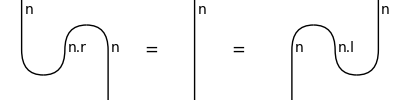# rigid#

The free rigid category, i.e. diagrams with cups and caps.

## Summary#

 `Ob` A rigid object has adjoints `Ob.l()` and `Ob.r()`. `Ty` A rigid type is a closed type with rigid objects inside. `PRO` A PRO is a natural number `n` seen as a rigid type with unnamed objects. `Diagram` A rigid diagram is a closed diagram with `Cup` and `Cap` boxes. `Box` A rigid box is a closed box in a rigid diagram. `Cup` The counit of the adjunction for an atomic type. `Cap` The unit of the adjunction for an atomic type. `Sum` A rigid sum is a closed sum that can be transposed. `Category` A rigid category is a monoidal category with methods `l`, `r`, `cups` and `caps`. `Functor` A rigid functor is a closed functor that preserves cups and caps.

## Axioms#

```>>> unit, s, n = Ty(), Ty('s'), Ty('n')
>>> t = n.r @ s @ n.l
>>> assert t @ unit == t == unit @ t
>>> assert t.l.r == t == t.r.l
>>> left_snake, right_snake = Id(n.r).transpose(left=True), Id(n.l).transpose()
>>> assert left_snake.normal_form() == Id(n) == right_snake.normal_form()
```
```>>> from discopy.drawing import Equation
>>> Equation(left_snake, Id(n), right_snake).draw(
...     figsize=(4, 2), path='docs/_static/rigid/typed-snake-equation.png')
```